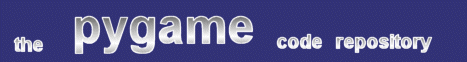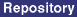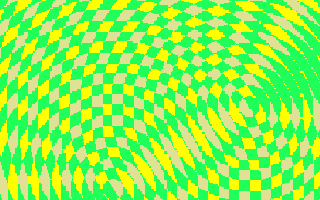### Title: Interference Pattern Effect

Author: Gareth Noyce (http://www.korruptor.demon.co.uk)
Submission date: July 11, 2002

Description: An effect that animates two copies of a concentric circle image to produce a nice interference effect.pygame version required: 1.3.4 with Surfarray
SDL version required: Any
Python version required: 2.0 with Numeric

Comments: From the "frigging-clever" department comes interference.py, Gareth Noyce's latest Numeric-powered submission. Once two-color gif image is used, and Numeric is used to slice the image into screen-sized sections. These sections are then superimposed using Numeric's very fast add() function, and the result is a three-color image that can be redrawn very rapdily to produce a nice effect. This script is a good example of Numeric used very well - slow for-loops are replaced by very fast slicing operations.

```
"""
Program		: interference.py
Author		: Gareth "Korruptor" Noyce -- http://www.korruptor.demon.co.uk
Desc		:

Yakk, Yakk. Interference Circles, more for the Amiga retro collection... :)

We load an image of concentric circles, whose dimensions are larger than the screen. Using
2 copies of the surfarray of this image; we take screen sized array-slices from each, moving
the offsets around a sin.  As the loaded image is a 2 colour gif, and the effect runs on an 8bit
display, we can use Numeric's add() to sum the arrays, giving a simple surfarray to a custom
3 colour palette. Nice...

Play with the colours, change the image loaded (I was lazy by making a gif, but it does allow you
to play around with different numbers of circles or random shapes...) and adjust the sines to suit
"""

import pygame, pygame.image
from pygame.surfarray import *
from pygame.locals import *
from Numeric import *

# ------------------------------------------------------------------------------------

RES 		= (320,200)
PI 		= 3.14159

# ------------------------------------------------------------------------------------
def main():

# Initialise pygame, and grab an 8bit display.
pygame.init()
screen		= pygame.display.set_mode(RES,0, 8)

# Setup the palette -- choice of colours is pretty important to getting the effect
# to work well. Mine aren't that great, but then, this is a quickie ;-)
colour_map = zeros((256, 3))

# Background colour...
colour_map =255
colour_map =255
colour_map =0

# Circles, not overlapping...
colour_map =32
colour_map =255
colour_map =89

# Circles, overlapping...
colour_map =225
colour_map =225
colour_map =148

screen.set_palette(colour_map)

# Something to write to...
screen_buffer	= pygame.surfarray.array2d(screen)

# Load the Base image and make a couple of copies...
ring1_arr 	= pygame.surfarray.array2d(ring1_surf)
ring2_arr	= ring1_arr

xang = 0
yang = 0
xang1 = 0
yang1 = 0

x = 0
y = 0
x1 = 0
y1 = 0

# Fruity loops...
while 1:

# Have we received an event to quit the program?
for e in pygame.event.get():
if e.type in (QUIT,KEYDOWN,MOUSEBUTTONDOWN):
return

# Calculate the offsets for the slices of the two images...
offx = ((RES/18)-10)*sin((xang*DEG2RAD) * 2.7)
offy = ((RES/13)-10)*cos((yang*DEG2RAD) * 2.5)
offx1 = ((RES/19)-12)*sin((xang1*DEG2RAD) * 2.2)
offy1 = ((RES/18)-12)*cos((yang1*DEG2RAD) * 2.5)

# Increment the positions of the slices...
x += offx
y += offy
x1 += offx1
y1 += offy1

xang += 1.2
yang += 2.1
xang1 += 1.5
yang1 += 1.7

# Cut each slice from it's surfarray: [bp+offset:bp+offset+width, bp+offset:bp+offset+height]
foo = ring1_arr[40+int(x):40+int(x)+RES, 200+int(y):200+int(y)+RES]
bar = ring2_arr[80+int(x1):80+int(x1)+RES, 240+int(y1):240+int(y1)+RES]

# Add the two arrays...

# Show results...
blit_array(screen, screen_buffer)
pygame.display.flip()

# Bye!
print screen_buffer
return

# ------------------------------------------------------------------------------------
if __name__ == '__main__': main()
# End of sauce. Pass the chips...

```
 From: hgrehgv Date: November 29, 2004 14:57 GMT

 Feedback